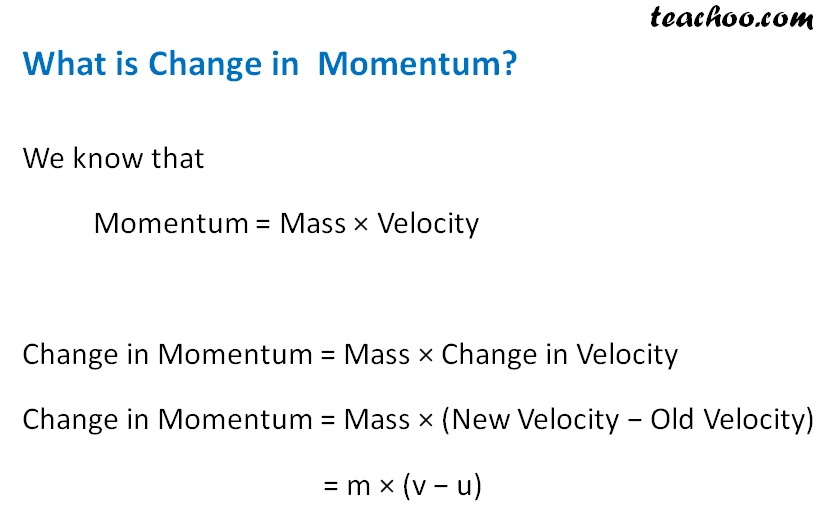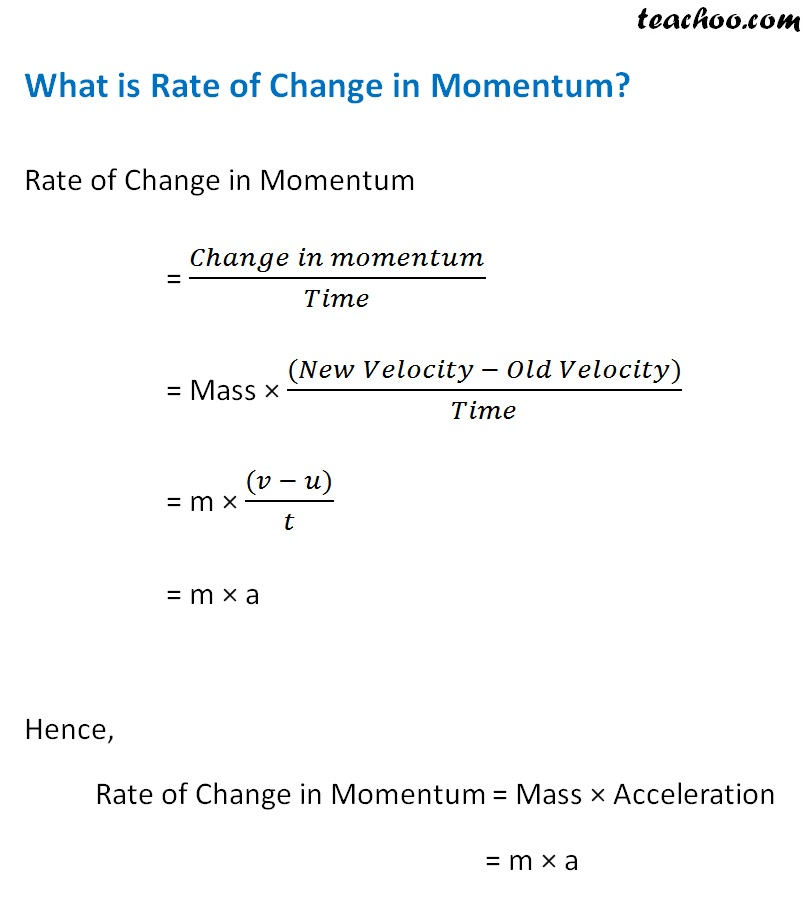Concepts

Class 9
Chapter 9 Class 9 - Force and Laws Of Motion

It is quantity of motion of a moving body

It is a product of mass and velocity

Momentum = Mass × Velocity

Momentum = m × v

Example

Suppose a person has a mass of 60 kg

He moves at a speed of 3 meters per second

What is Momentum

Mass = m = 60 kg

Velocity = v = 3 m/s

Momentum = Mass x Velocity

= 60 kg x 3 m/s

= 180 kg m/s

What is Unit of Momentum

It is kilogram meter per second or kg m/s

Explanation

We know that

Momentum = Mass x Velocity

Mass is measured in kg and Velocity in meter/second

So Momentum-kg m/s

## Whose Momentum will be Higher-Heavy object or Light Object?

Momentum of heavier object will be higher

Since momentum is a product of Mass and Velocity

We can change momentum by increasing mass of object

Example 1

Suppose 2 balls are thrown at same velocity of 10 meters/second

One is tennis ball of 100 grams (0.1 kg) other is cricket ball of 600 grams (0.6 kg0

Which has higher momentum

Cricket ball will have higher momentum because it has higher mass

Explanation

Momentum of Tennis ball

= Mass x Velocity

= 0.1 x 10

=1 kg m/s

Momentum of Cricket ball

= Mass x Velocity

= 0.6 x 10

= 6 kg m/s

Momentum of Cricket bal is higher due to its higher mass

## Whose Momentum is Higher-An object at Higher Speed or Lower Velocity?

Momentum of object moving at higher velocity will be higher

Since momentum is a product of Mass and Velocity

We can change momentum by increasing velocity of object

Example

A ball bowled by fast bowler hits a batsman and hurts him

But if same ball is bowled by a slow bowler hits a batsman it does not hurt

Why

Ans

It is because of higher momentum in case of fast ball

Explanation

In this case, mass of ball is same in both the case

However, velocity of ball is more in case it is bowled by fast bowler

So, momentum will also be more## Questions

NCERT Question 9 - What is the momentum of an object of mass m, moving with a velocity v?
(a) (mv) 2 (b) mv 2 (c) ½ mv 2 (d) mv

NCERT Question 13 - A hockey ball of mass 200 g travelling at 10 m s–1 is struck by a hockey stick so as to return it along its original path with a velocity at 5 m s–1. Calculate the magnitude of change of momentum occurred in the motion of the hockey ball by the force applied by the hockey stick.

NCERT Question 18 - How much momentum will a dumbbell of mass 10 kg transfer to the floor if it falls from a height of 80 cm? Take its downward acceleration to be 10 m s–2.

Learn in your speed, with individual attention - Teachoo Maths 1-on-1 Class

### Transcript

What is Change in Momentum? We know that Momentum = Mass × Velocity Change in Momentum = Mass × Change in Velocity Change in Momentum = Mass × (New Velocity − Old Velocity) = m × (v − u) What is Rate of Change in Momentum? Rate of Change in Momentum = (𝐶ℎ𝑎𝑛𝑔𝑒 𝑖𝑛 𝑚𝑜𝑚𝑒𝑛𝑡𝑢𝑚)/𝑇𝑖𝑚𝑒 = Mass × ((𝑁𝑒𝑤 𝑉𝑒𝑙𝑜𝑐𝑖𝑡𝑦 − 𝑂𝑙𝑑 𝑉𝑒𝑙𝑜𝑐𝑖𝑡𝑦))/𝑇𝑖𝑚𝑒 = m × ((𝑣 − 𝑢))/𝑡 = m × a Hence, Rate of Change in Momentum = Mass × Acceleration = m × a